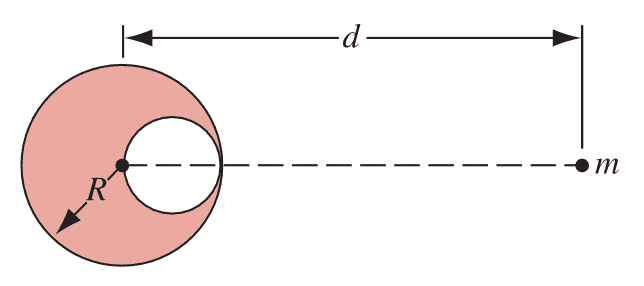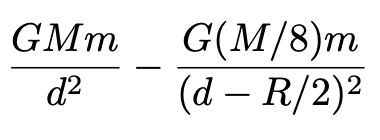# Hollowed out sphere exerting gravitational force

jolly_math
Homework Statement:
A spherical hollow is made in a lead sphere of radius R, such that its surface touches the outside surface of the lead sphere and passes through its centre. The mass of the sphere before hollowing was M. With what force, according to the law of universal gravitation, will the hollowed lead sphere attract a small sphere of mass m, which lies at a distance d from the centre of the lead sphere on the straight line connecting the centres of the spheres and of the hollow?
(diagram below)
Relevant Equations:
F = G*m1m2/rI solved that the hollowed out mass is M/8, which is correct. I don't understand why it is incorrect to substitute the remaining mass (7M/8) back into the F = G*m1m2/r to produce the force. Why is the solution the force of the whole lead sphere minus the force of the “hole” lead sphere, which is? What effect does this "hole" have?

Thank you.

Homework Helper
Gold Member
This idea is superposition. The force at mass ##m## due to a full sphere is greater than that due to the hollowed sphere because there is more mass. To get the force due to the hollowed sphere, you need to subtract the force due to the smaller sphere that you took out in order to create the cavity.

•PhDeezNutz and PeroK
jolly_math
This idea is superposition. The force at mass ##m## due to a full sphere is greater than that due to the hollowed sphere because there is more mass. To get the force due to the hollowed sphere, you need to subtract the force due to the smaller sphere that you took out in order to create the cavity.
Is the force needed to create the cavity a gravitational force?

•BvU
Homework Helper
No, pure elbow grease ...I don't understand why it is incorrect to substitute the remaining mass (7M/8) back into the F =
Because the remaining mass doesn't have its center of mass at the same position !

##\ ##

•PeroK, jbriggs444, erobz and 1 other person
•